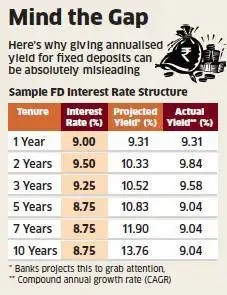# ATM :: Banks should show real yield on fixed deposits

The main problem lies in the fact that what banks are projecting is the simple interest and not the compound annual growth rate.
By Narendra Nathan, ET Bureau | 22 Oct, 2013, 11.45AM IST | Economic TimesMUMBAI: Recently, the Reserve Bank of India has taken steps to increase the level of transparency in bank loans. For example, RBI has restricted banks from charging higher interest rates and at the same time projecting them as “zero interest loans”. However, banks continue to follow less transparent practice with other products and most important among them is the interest paid on fixed deposits (FDs). For example, the FD pamphlets from most banks usually carry two columns – one for the interest rate and other for the “annualised yield”. The explanation to the annualised yield column will also state that the “annualised yield is calculated on the basis of quarterly compounding for the entire tenure“.

Why is giving annualised yield bad, you may ask. The problem is not in giving the annualised yield, but in giving the wrong yield (i.e, much higher figures) to grab the attention of FD investors. For example, a bank offering an interest of 8.75% per annum for its 10-year deposits usually projects that the annualised yield for the same is as 13.76%. Though the actual yield will be slightly higher due to quarterly compounding, the compound annual growth rate (CAGR) for a 10-year FD will only be 9.04% and not 13.76% as projected by the bank.

Now, let us take a closer look at how these banks arrive at this 13.76% figure for the 10-year FDs. To make the computation easier, let us assume that the amount invested in the 10-year FD is Rs 1 lakh. Since the FD is compounded quarterly, 2.19% (i.e, 1/4 of 8.75%) of Rs 1 lakh is added at the end of first quarter. So the value at the end of first quarter goes up to 102,190. Similarly, 2.19% of 102,190 is added at the end of second quarter and this process is continued till the end of 10 years. So the initial investment value of Rs 1 lakh grows to Rs 237, 635 at the end of 10 years. Since this is an absolute return of 137.64%, banks divide this value by 10 years to arrive at the 13.76% yield.Since the above mentioned computation is correct, what is the problem, you may ask. The main problem lies in the fact that what they are projecting is the simple interest and not the compound annual growth rate. For example, the CAGR of an investment that has generated an absolute return of 300% in 10 years is just 14.87% and not 30% per annum. CAGR is the best way to compare return rates between two financial products and that explains why Sebi has made it mandatory for mutual funds to use CAGR while giving their historical returns.

Source : http://goo.gl/obpdcR